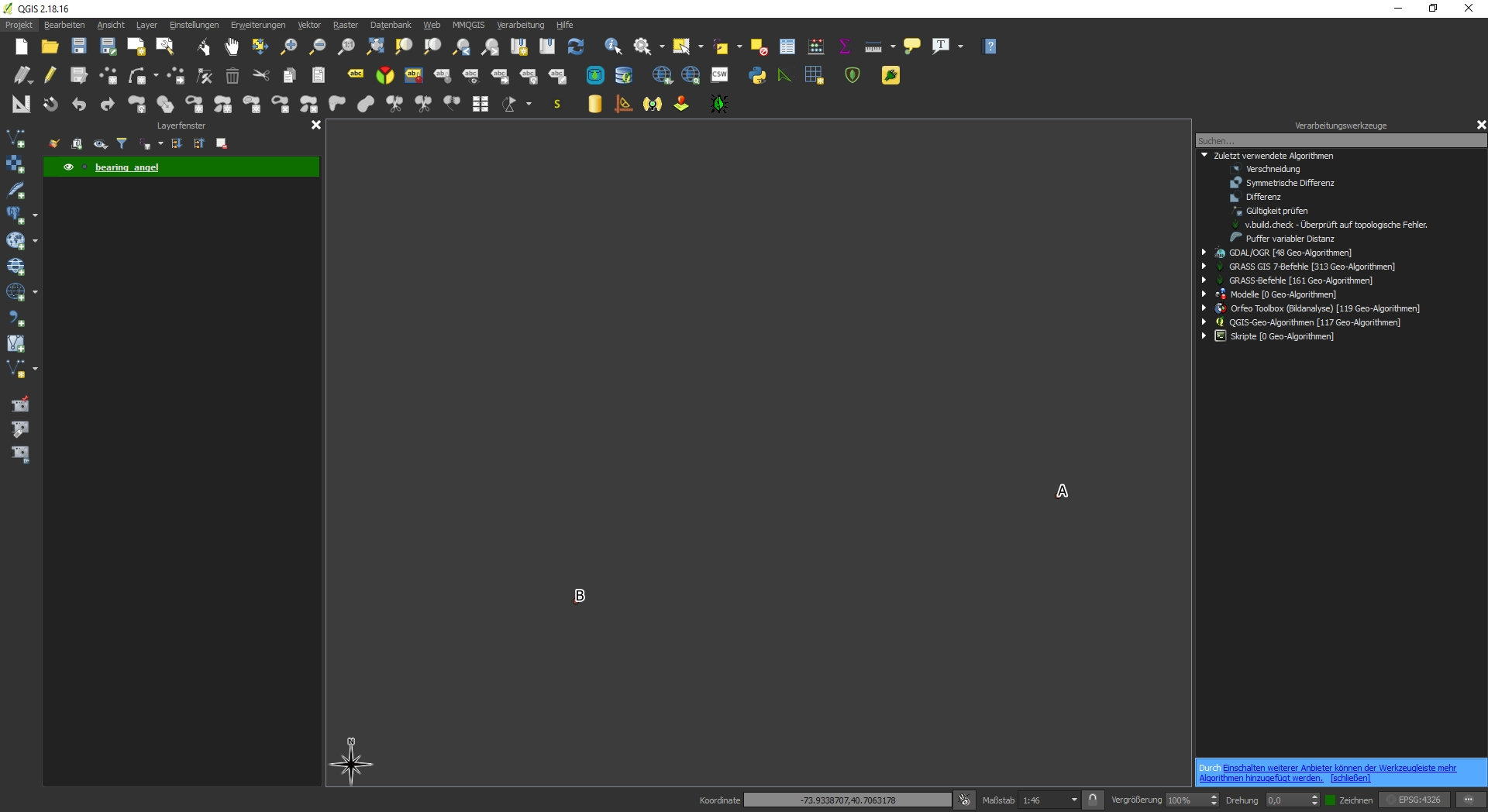Instantly share code, notes, and snippets.jeromer/compassbearing.py Last active Oct 16, 2019

compass bearing between two points in Python
 # LICENSE: public domain def calculate_initial_compass_bearing(pointA, pointB): """ Calculates the bearing between two points. The formulae used is the following: θ = atan2(sin(Δlong).cos(lat2), cos(lat1).sin(lat2) − sin(lat1).cos(lat2).cos(Δlong)) :Parameters: - `pointA: The tuple representing the latitude/longitude for the first point. Latitude and longitude must be in decimal degrees - `pointB: The tuple representing the latitude/longitude for the second point. Latitude and longitude must be in decimal degrees :Returns: The bearing in degrees :Returns Type: float """ if (type(pointA) != tuple) or (type(pointB) != tuple): raise TypeError("Only tuples are supported as arguments") lat1 = math.radians(pointA) lat2 = math.radians(pointB) diffLong = math.radians(pointB - pointA) x = math.sin(diffLong) * math.cos(lat2) y = math.cos(lat1) * math.sin(lat2) - (math.sin(lat1) * math.cos(lat2) * math.cos(diffLong)) initial_bearing = math.atan2(x, y) # Now we have the initial bearing but math.atan2 return values # from -180° to + 180° which is not what we want for a compass bearing # The solution is to normalize the initial bearing as shown below initial_bearing = math.degrees(initial_bearing) compass_bearing = (initial_bearing + 360) % 360 return compass_bearing

jakebathman commented Jun 5, 2013

 Exactly what I was looking for. Nice work.

ehippy commented Mar 9, 2014

 Likewise! This was perfect!

yinzhelu commented Nov 29, 2014

 Thanks. This is perfect for my pet project.

sgzu commented May 5, 2015

 Thank you!

greasysock commented Oct 27, 2015

 I was wondering why my ships in ksp were never exactly on target, this is great!

douglasgoodwin commented Dec 12, 2015

 thanks -- this is much better than what I was using before!

jligeza commented Apr 13, 2016

 At last an algorithm that just works. Thank you.

pavanKavvuri commented Mar 5, 2017

 Great {Pro}

josh-marasigan commented Apr 12, 2017

 This works great! Thank you.

pedrocamargo commented Apr 17, 2017

 Awesome!

quieterkali commented Apr 17, 2017 • edited

 Thank you very much 🥇

pedrocamargo commented Apr 23, 2017

 What is the license for this code, @jeromer?

 up !

fredvollmer commented May 26, 2017

 Very nice, especially all the explanation. Thank you!

dali5 commented Jul 19, 2017

 thank you

mapcloud commented Jul 27, 2017

 Thank you for sharing!

 great

glaucomunsberg commented Oct 13, 2017

 Great!!

echocodepy commented Nov 17, 2017

 awesome work man

ocean86 commented Nov 20, 2017

 Thanks!

falcon78 commented Nov 23, 2017

 Holy shit bro , this code is fucking awesome. Thx a lot man !

zaarcvon commented Feb 9, 2018

 Thank you for sharing!

blackgis commented Feb 22, 2018 • edited

 I have an issue with that, the output is different then expected: A = (-73.933781942,40.7062912231) B = (-73.93390547,40.7062643692) bearing is 183.44288775507985 but it should be nearly 270 when BA: bearing is 3.4429135601084795 but it should be almost 90 could it be that there is a wired 90 degree changing required somewhere?Update yes @schicks you are right, i messed up the x and y and i wasn't aware that i can use the wgs84 coordinates without transforming to a 2D Now it gives back 253.99863197083152 what is expected

schicks commented Feb 27, 2018

 @blackgis, I think you may have the arguments a little messed up. They should be (lat, lon), not (lon, lat).

lionsterben commented Apr 24, 2018

 thank you!

Amytipple commented May 25, 2018

 Thanks!

Gurpmond commented Jun 27, 2018 • edited

 import math lat1 = math.radians(float(input("Lattitude1:"))) lat2 = math.radians(float(input("Lattitude2:"))) long1 = math.radians(float(input("Longitude1:"))) long2 = math.radians(float(input("Longitude2:"))) longdifference = math.radians(float(long2-long1)) x = math.sin(longdifference) * math.cos(lat2) y = math.cos(lat1) * math.sin(lat2) - (math.sin(lat1)* math.cos(lat2) * math.cos(longdifference)) initial_bearing = math.atan2(x, y) initial_bearing = math.degrees(initial_bearing) compass_bearing = (initial_bearing + 360) % 360 print(initial_bearing, "°") Output: Lattitude1:47.5606 Lattitude2:47.594719 Longitude1:-52.743099 Longitude2:-52.685001 Result: 1.1481674985452277 ° this should be 49°, why i am getting wrong value ?

tboutaour commented Jul 9, 2019

 Great work! It's what I looking for. Thank you

nikisix commented Aug 22, 2019

 Good job!
to join this conversation on GitHub. Already have an account? Sign in to comment
You can’t perform that action at this time.